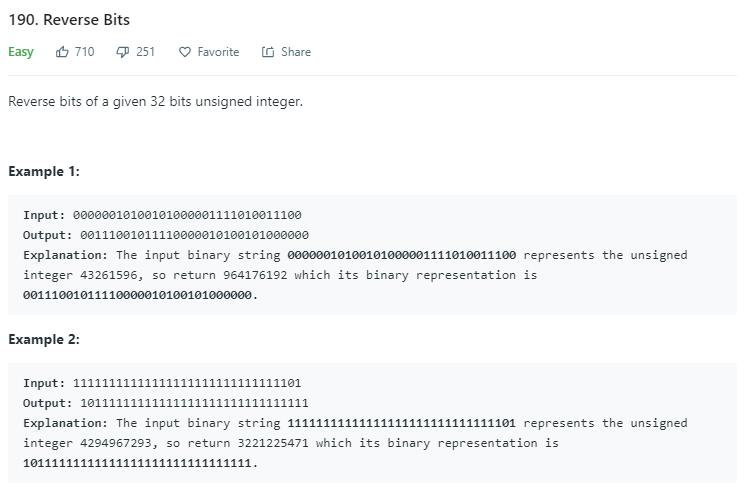# 题目描述（简单难度）# 解法一

原数字 1011 ，res = 0

res 左移一位，res = 0，

1011 右移一位变为 101

res = 1 左移一位，res = 10，

101 右移一位变为 10

res = 11 左移一位，res = 110，

10 右移一位变为 1

res = 110 左移一位，res = 1100，

1 右移一位变为 0, 结束


public int reverseBits(int n) {
int res = 0;
int count = 0;
while (count < 32) {
res <<= 1;  //res 左移一位空出位置
res |= (n & 1); //得到的最低位加过来
n >>= 1;//原数字右移一位去掉已经处理过的最低位
count++;
}
return res;
}


# 解法二

(1100) & x >>> 2 | (0011) & x <<< 2 = (0011)|(0100)= 0111，然后就完成了 1101 的交换。

public int reverseBits(int n) {
n = ((n & 0xffff0000) >>> 16) | ((n & 0x0000ffff) << 16);
n = ((n & 0xff00ff00) >>> 8) | ((n & 0x00ff00ff) << 8);
n = ((n & 0xf0f0f0f0) >>> 4) | ((n & 0x0f0f0f0f) << 4);
n = ((n & 0xcccccccc) >>> 2) | ((n & 0x33333333) << 2);
n = ((n & 0xaaaaaaaa) >>> 1) | ((n & 0x55555555) << 1);
return n;
}